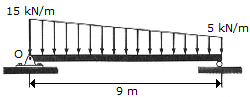# Engineering Mechanics - Force System Resultants - Discussion

### Discussion :: Force System Resultants - General Questions (Q.No.9)

9.Replace the loading by an equivalent force and couple moment acting at point O.

 [A]. R = 90 kN, M = 473 kN-m CW [B]. R = 90 kN, M = 338 kN-m CW [C]. R = 45 kN, M = 203 kN-m CW [D]. R = 135 kN, M = 270 kN-m CW

Explanation:

No answer description available for this question.

 Md Salahuddin said: (Dec 19, 2017) Trapezoidal load =1/2(a+b)*h, 1/2(15+5)*9=1/2*20*9=10*9=90kN.

 Md Salahuddin said: (Dec 19, 2017) Trapezoidal load =1/2(a+b)*h, 1/2(15+5)*9=1/2*20*9=10*9=90kN.